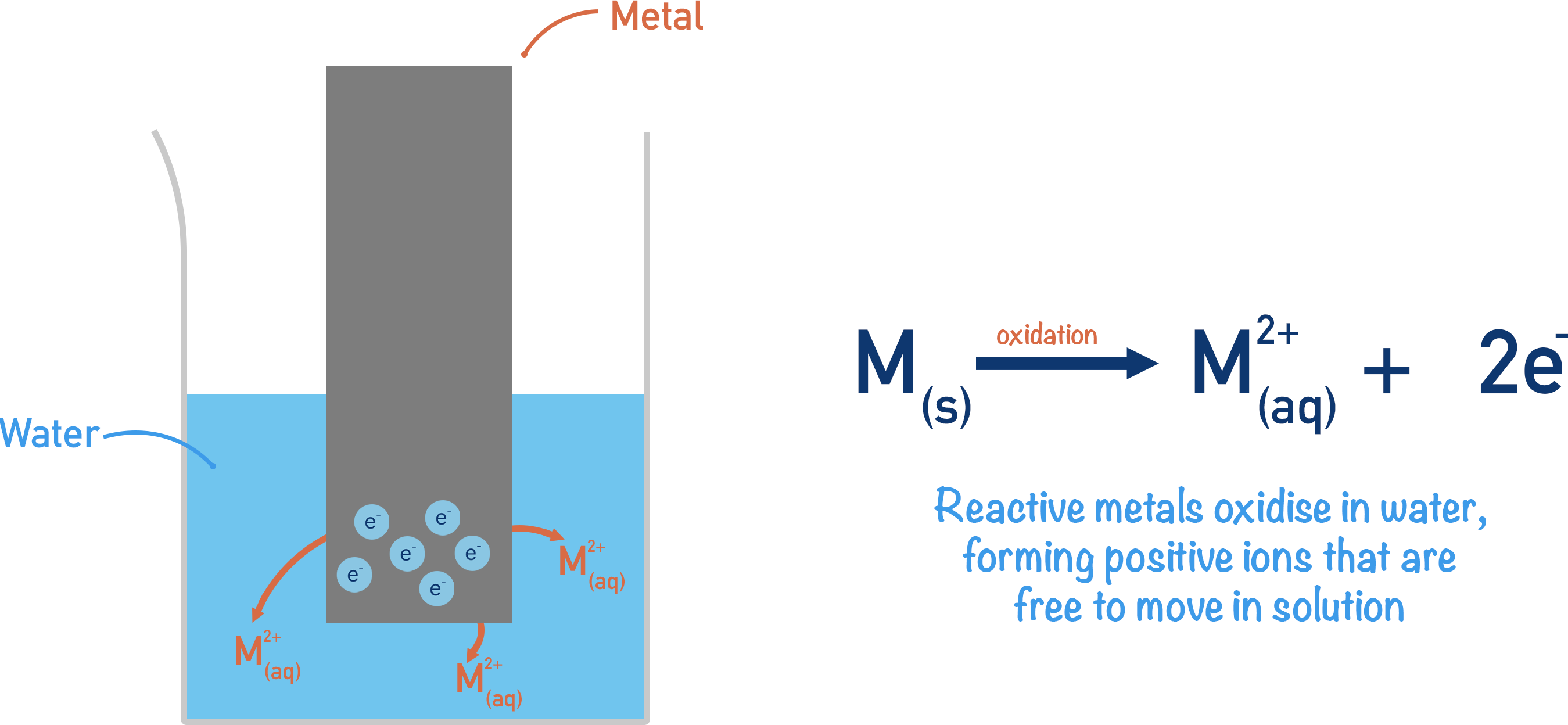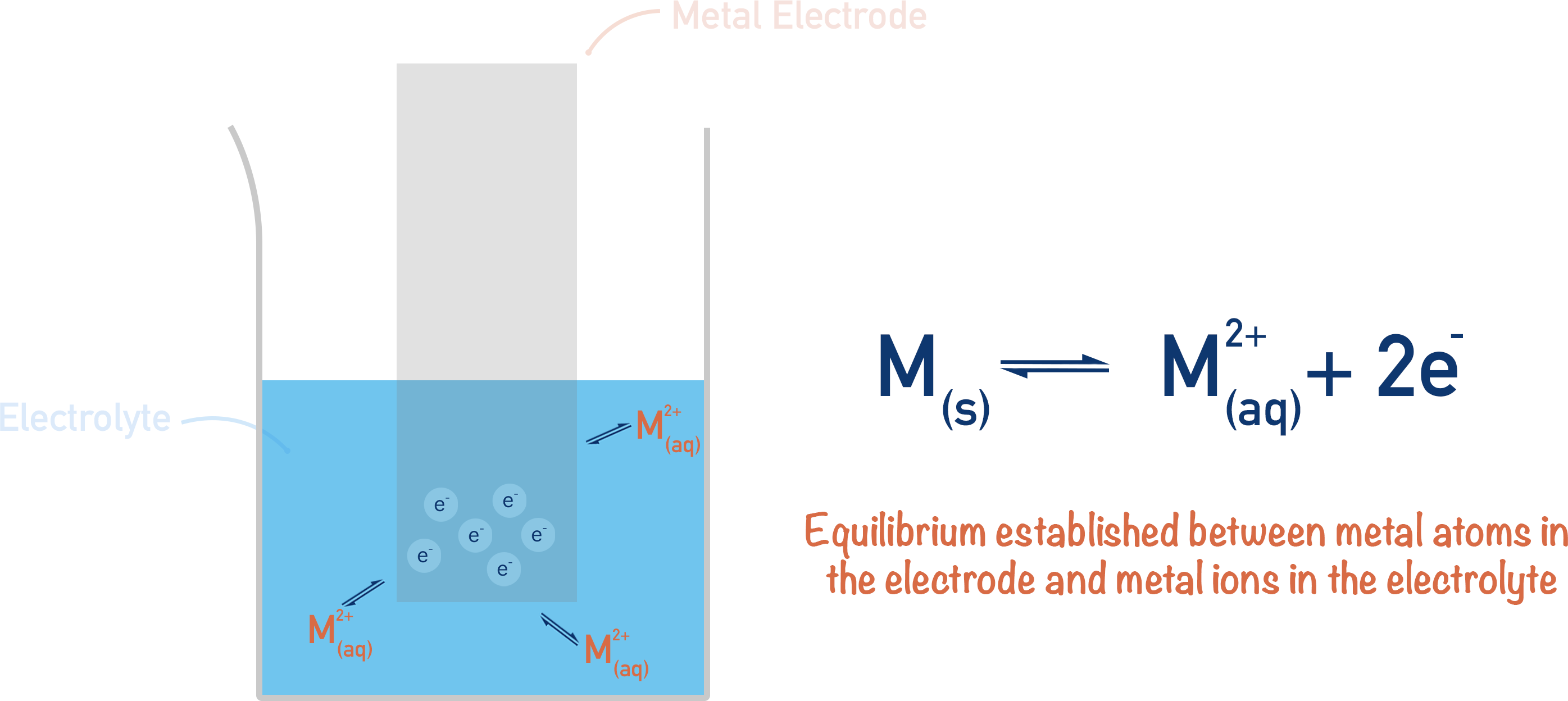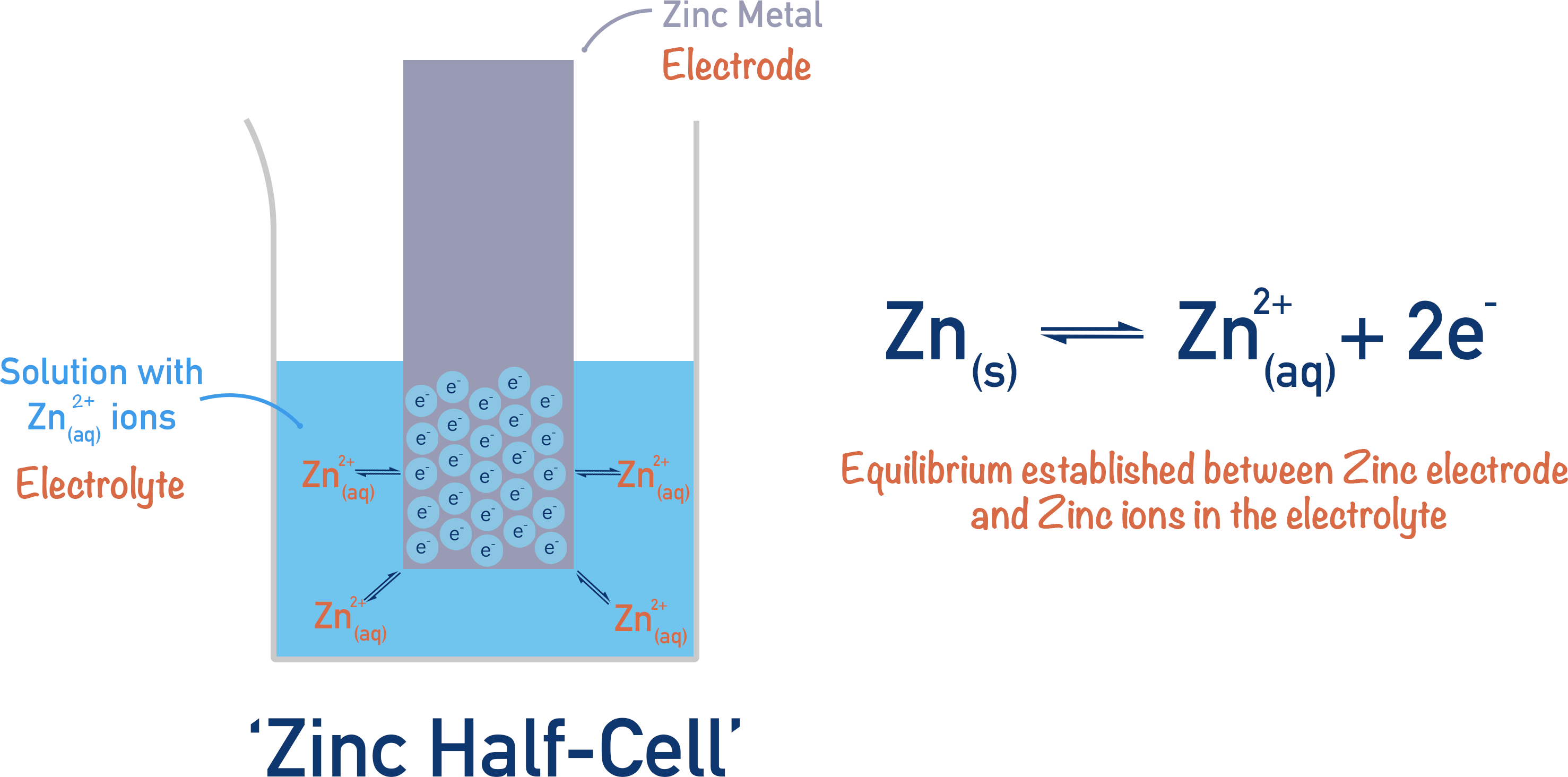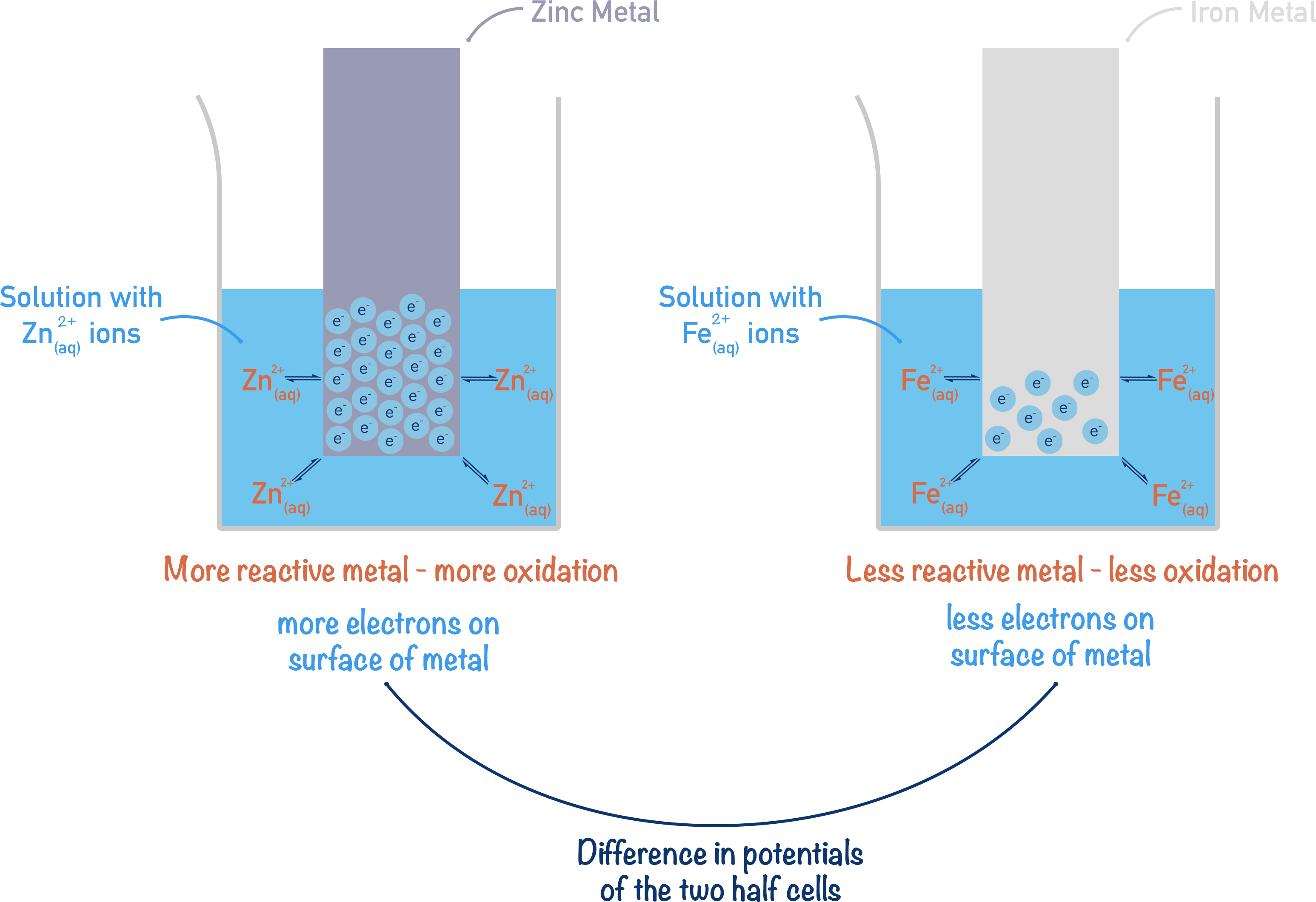Quick Notes Electrochemistry - The Basics

• Metals react by losing electrons and forming positively charged ions, this is oxidation.
• The reactivity of a metal refers to how easily it can lose electrons (and form positively charged ions).
• Electrodes are solid surfaces that, when in solution, can ‘hold’ electrons on their surface or give electrons away.
• Simple half-cells are made of a metal electrode placed into an electrolyte, which contains ions of the metal used for the electrode.
• When a reactive metal electrode is placed in an electrolyte containing ions of itself, an equilibrium is established between the ions in the electrolyte and the metal electrode.
• A build-up of electrons on an electrode’s surface creates an electrical potential.
• Charged ions cause the electrolyte to have an electrical potential.
• The electrical potential of the electrode and the electrical potential of the electrolyte are different – creating a potential difference between the two – this is called an electrode potential.
• Different metals have different electrode potentials based on their reactivities.

Full Notes Electrochemistry - The Basics

Electrochemistry is the combined study of chemical reactions and electricity. There are two ways chemical reactions and electricity can interact. Electricity can be used to force certain chemical reactions to happen, or certain chemical reactions can be used to generate electricity.

It is important to define electricity here. Very simply, ‘electricity’ (electrical current) is the ‘net movement of charge’. It doesn’t matter what form the charge is in (as in negatively charged electrons or positively charged ions), if there is a net movement of charged particles in a direction, an electrical current is present.

Once the basic principles are understood, electrochemistry can be ‘relatively’ straightforward, and post A-level it can be used to explain a whole range of chemical reactions! These notes may seem long, but it’s vital that the basics are fully understood before attempting exam questions and revising.

## The basics

When metals react, they are oxidised – their atoms lose electrons to become positively charged ions. Different metals oxidise more easily than others, making them more reactive. A highly reactive metal (potassium, for example) easily loses electrons to form positively charged ions, whereas an unreactive metal (gold, for example) doesn’t lose electrons and no ions form.

When a reactive metal is placed in water, some of its atoms are oxidised – losing electrons to form positively charged ions.The positively charged ions dissolve into the water. The electrons, however, have nowhere to go and build-up on the surface of the solid metal.

Any build-up of charge on a surface is described as an ‘electrical potential’. The piece of metal now has an electrical potential, which (since electrons are negatively charged) is a negative electrical potential (compared to the electrolyte).

The solution also has an electrical potential due to the build-up of positively charged ions in the water. As the ions are positively charged, this electrical potential is positive (compared to the electrode surface).

Any solid object in a solution that can ‘hold’ electrons on its surface or ‘give away’ electrons is called an electrode. The metal strip can be considered to be an electrode because it builds up electrons on its surface (acts as a sink of electrons). A solution that enables electricity to flow through it, or can hold an electrical potential, is called an ‘electrolyte’.Most metals react in the following way (although not all metals form a 2+ charge) when oxidised.

M(s) →M2+(aq) + 2e-

Oxidation of metals is a reversible process: positively charged metal ions can be reduced by gaining electrons.

M2+(aq) + 2e- →M(s)

Once there is a high enough concentration of positive ions in the solution, the metal ions will pick up electrons from the surface of the metal and reform solid metal atoms. The metal ions are reduced.Eventually, an equilibrium will form between the water with ions in (electrolyte) and the solid metal (electrode), meaning the electrical potentials of both will remain constant.

These two potentials will be different, meaning there is a potential difference between the metal strip (electrode) and the solution (electrolyte). This potential difference is called an ‘electrode potential’. As all metals have a different reactivity, they would all have a different electrode potential when placed in the same solution.

If you place a piece of metal in a solution that contains ions of that metal, equilibrium will be established much faster than when simply using water as above. The position of equilibrium can also be changed by altering the concentration of ions in the solution, forming a new electrode potential (potential difference between the metal strip and the solution). This arrangement is called a half-cell.

If there is a high concentration of metal ions in a solution, the position of equilibrium will move to the right; electrons will actually be removed from the surface of the metal (electrode) as more reduction of metal ions will happen. Now, the electrode potential will be different as it will be forced to give electrons to the metal ions for a reduction reaction.

How negative or positive the electrode potential is can be controlled by the reactivity of the metal electrode used and the concentration of its ions in the electrolyte used in the half-cell.The absolute key thing to understand here is that the position of equilibrium in the half-cell can be changed! This is important when dealing with electrochemical cells and cell potentials – this is to come!

## Comparing Half-cells

Take iron and zinc. Zinc is more reactive than iron, so if solid samples of both are placed in separate solutions containing their own ions, more zinc ions will be present than iron. To keep it simple, we will assume both solutions are the same concentration.If more Zn2+ ions are present, more electrons are on the surface of the metal. More electrons on the surface of the metal electrode means the electrode has a more negative potential.

There would be fewer electrons on the iron metal strip, so the iron electrode would have a potential that is not as negative as the zinc. This means compared to the zinc half-cell, the iron half-cell would have a positive electrode potential.

Electrochemistry is all about measuring how the electrode potential of one half-cell compares to the electrode potential of another half-cell, and how these values can be used to predict reactions. When two half-cells are connected together, an electrochemical cell is formed. .